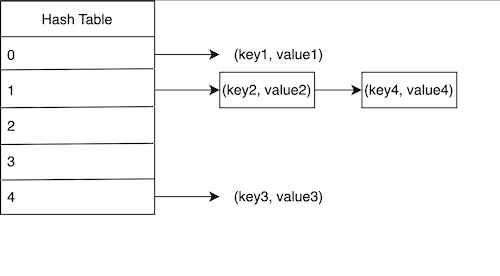# Hash Tables or Hash Maps

In simple words, it is a data structure for storing data, that is really efficient when fetching data from it.

## How does it store data?

There are many implementations possible for this data structure. But let’s see the very common and basic implementation of Hash tables.

Technically, a Hash Table is an Array. We insert key-value pairs in the array in the form of Tuples, i.e. (key, value).

Throughout this article, I may use the term Hash Table and Array interchangeably, because both of them are same.

But how do we know which key-value pair should be stored at which index of the array (or hash table)? Here comes the most important concept of Hash Table, Hashing.

Hashing is done using a hash function which calculates the integer value from the key. We should also take care that hashed value does not go beyond the size of the array (which would result in an index out of bounds). Therefore, we take the modulus of hashed value with the array’s size. This would give the index within the bounds of the array’s size, and we can easily store our key-value pair in that index. For example, please see below how hashing calculates the index of the hash table.

## Collisions

A collision happens when two or more keys have the same index in the hash table (or array). Two possible ways of getting a collision:

1. Two keys have the same hash value, which in turn have the same index.
2. Two keys have different hash values, but when taking their mod, they return the same index. Example: Hash values 273 and 183 will have the same index when the array size is 10.

To avoid a collision, instead of storing key-value pairs directly in the array, we use chaining (or pointer) from the index of the array. Each index can contain a linked list or an array of key-value pairs.A hash table of capacity 5 where each element is containing array of key-value pairs

Cheers…!

--

--